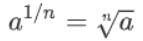# Eight Rules of Exponents and Calculator#Laws of Exponents | Golden Rules of Exponents

An online exponents rule

Law Name Law Example Rule
One Rule x1 = x 91 = 9 Any number raised to the power of "1" equals itself.
Zero Rule x0 = 1 70 = 1 Any non-zero number raised to the power of zero equals 1
Negative Exponent x-1 = 1/x 4-1 = 1/4 Any non-zero number raised to a negative power equals its reciprocal raised to the opposite positive power.
Product Rule xmxn = xm+n x2x3 = x2+3 = x5 When multiplying 2 powers that have the same base, you can add the exponents.
Quotient Rule xm/xn = xm-n x6/x2 = x6-2 = x4 Divide 2 powers with the same base by subtracting the exponents
Power Rule (xm)n = xmn (x2)3 = x2×3 = x6 Multiply the exponents
Tower Rule anm 222 = 24 = 16 Multiply the exponents from the top down.
Fraction RuleSum can be rewritten using radicals.Denominator of the fraction becomes the index of the radical.# Laws of Exponents Calculator

##Age Calculators ►

Horse Age Calculator , Cow Age Calculator , Cat Age Calculator , Dog Age Calculator, Human Age Calculator 100 Days Old Calculator Date Difference Calculator